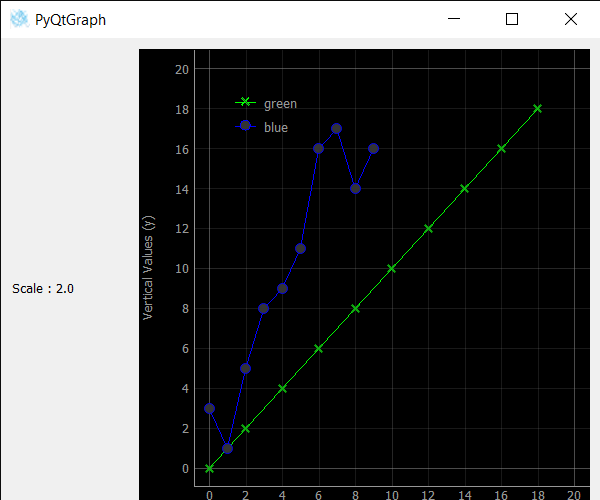Open in App
Not now

# PyQtGraph – Getting Scale Factor of Line in Line Graph

• Last Updated : 20 Jan, 2022

In this article we will see how we can get the scale factor of the line of line graph in the PyQtGraph module. PyQtGraph is a graphics and user interface library for Python that provides functionality commonly required in designing and science applications. Its primary goals are to provide fast, interactive graphics for displaying data (plots, video, etc.) A line chart or line plot or line graph or curve chart is a type of chart that displays information as a series of data points called ‘markers’ connected by straight line segments. It is a basic type of chart common in many fields. The line graph is created with the help of plot class in PyQtGraph. The scale factor is the factor that is used as the factor by which data of line is multiplied. It can be set with the help of setScale method.
We can create a plot window and create lines on it with the help of commands given below

```# creating a pyqtgraph plot window
plt = pg.plot()

# plotting line in green color
# with dot symbol as x, not a mandatory field
line = plt.plot(x, y, pen='g', symbol='x', symbolPen='g',
symbolBrush=0.2, name='green')```

In order to do this we use scale method with the line object
Syntax : line.scale()
Argument : It takes no argument
Return : It returns float value

Below is the implementation

## Python3

 `# importing Qt widgets` `from` `PyQt5.QtWidgets ``import` `*` `import` `sys`   `# importing pyqtgraph as pg` `import` `pyqtgraph as pg` `from` `PyQt5.QtGui ``import` `*`   `# Bar Graph class` `class` `BarGraphItem(pg.BarGraphItem):`   `    ``# constructor which inherit original` `    ``# BarGraphItem` `    ``def` `__init__(``self``, ``*``args, ``*``*``kwargs):` `        ``pg.BarGraphItem.__init__(``self``, ``*``args, ``*``*``kwargs)`   `    ``# creating a mouse double click event` `    ``def` `mouseDoubleClickEvent(``self``, e):`   `        ``# setting scale` `        ``self``.setScale(``0.2``)`     `class` `Window(QMainWindow):`   `    ``def` `__init__(``self``):` `        ``super``().__init__()`   `        ``# setting title` `        ``self``.setWindowTitle(``"PyQtGraph"``)`   `        ``# setting geometry` `        ``self``.setGeometry(``100``, ``100``, ``600``, ``500``)`   `        ``# icon` `        ``icon ``=` `QIcon(``"skin.png"``)`   `        ``# setting icon to the window` `        ``self``.setWindowIcon(icon)`   `        ``# calling method` `        ``self``.UiComponents()`   `        ``# showing all the widgets` `        ``self``.show()`   `    ``# method for components` `    ``def` `UiComponents(``self``):`   `        ``# creating a widget object` `        ``widget ``=` `QWidget()`   `        ``# creating a new label` `        ``label ``=` `QLabel(``"GeeksforGeeks Line Plot"``)`   `        ``# making it multiline` `        ``label.setWordWrap(``True``)`   `        ``# y values to plot by line 1` `        ``y ``=` `[``0``, ``1``, ``2``, ``3``, ``4``, ``5``, ``6``, ``7``, ``8``, ``9``]`   `        ``# y values to plot by line 2` `        ``y2 ``=` `[``3``, ``1``, ``5``, ``8``, ``9``, ``11``, ``16``, ``17``, ``14``, ``16``]` `        ``x ``=` `range``(``0``, ``10``)`   `        ``# create plot window object` `        ``plt ``=` `pg.plot()`   `        ``# showing x and y grids` `        ``plt.showGrid(x``=``True``, y``=``True``)`   `        ``# adding legend` `        ``plt.addLegend()`   `        ``# set properties of the label for y axis` `        ``plt.setLabel(``'left'``, ``'Vertical Values'``, units``=``'y'``)`   `        ``# set properties of the label for x axis` `        ``plt.setLabel(``'bottom'``, ``'Horizontal Values'``, units``=``'s'``)`   `        ``# setting horizontal range` `        ``plt.setXRange(``0``, ``20``)`   `        ``# setting vertical range` `        ``plt.setYRange(``0``, ``20``)`   `        ``# plotting line in green color` `        ``# with dot symbol as x, not a mandatory field` `        ``line1 ``=` `plt.plot(x, y, pen``=``'g'``, symbol``=``'x'``,` `                         ``symbolPen``=``'g'``, symbolBrush``=``0.2``, name``=``'green'``)`   `        ``# plotting line2 with blue color` `        ``# with dot symbol as o` `        ``line2 ``=` `plt.plot(x, y2, pen``=``'b'``, symbol``=``'o'``,` `                         ``symbolPen``=``'b'``, symbolBrush``=``0.2``, name``=``'blue'``)`   `        ``# setting scale of line 1` `        ``line1.setScale(``2``)`   `        ``# getting scale value  of line 1` `        ``value ``=` `line1.scale()`   `        ``# setting text to the label` `        ``label.setText(``"Scale : "` `+` `str``(value))`   `        ``# label minimum width` `        ``label.setMinimumWidth(``120``)`   `        ``# Creating a grid layout` `        ``layout ``=` `QGridLayout()`   `        ``# setting this layout to the widget` `        ``widget.setLayout(layout)`   `        ``# adding label to the layout` `        ``layout.addWidget(label, ``1``, ``0``)`   `        ``# plot window goes on right side, spanning 3 rows` `        ``layout.addWidget(plt, ``0``, ``1``, ``3``, ``1``)`   `        ``# setting this widget as central widget of the main window` `        ``self``.setCentralWidget(widget)`   `# create pyqt5 app` `App ``=` `QApplication(sys.argv)`   `# create the instance of our Window` `window ``=` `Window()`   `# start the app` `sys.exit(App.``exec``())`

Output :My Personal Notes arrow_drop_up
Related Articles